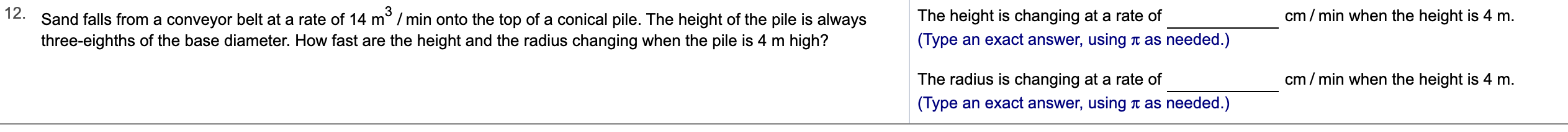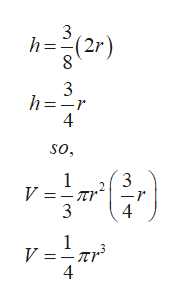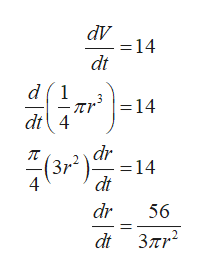# 12.Sand falls from a conveyor belt at a rate of 14 m2 /min onto the top of a conical pile. The height of the pile is alwayscm min when the height is 4 mThe height is changing at a rate of(Type an exact answer, using t as needed.)three-eighths of the base diameter. How fast are the height and the radius changing when the pile is 4 m high?cm/min when the height is 4 m.The radius is changing at a rate of(Type an exact answer, usingas needed.)

Question
34 views

Can you help me step by step?help_outlineImage Transcriptionclose12. Sand falls from a conveyor belt at a rate of 14 m2 /min onto the top of a conical pile. The height of the pile is always cm min when the height is 4 m The height is changing at a rate of (Type an exact answer, using t as needed.) three-eighths of the base diameter. How fast are the height and the radius changing when the pile is 4 m high? cm/min when the height is 4 m. The radius is changing at a rate of (Type an exact answer, using as needed.) fullscreen
check_circle

Step 1

Volume of a conical pile having radius r and height h is given by:

Step 2

And it is given that height of pile is three-eighths of diameter that is,help_outlineImage Transcriptionclose3 (2r) 3 h -r 4 so, 3 V =-nr 3 1 4 1 V = 4 fullscreen
Step 3

Now, since the sand is falling at a rate of 1...help_outlineImage TranscriptionclosedV - = 14 dt d1 = 14 dt4 dr (3r2 - = 14 dt dr 56 dt 37mr2 fullscreen

### Want to see the full answer?

See Solution

#### Want to see this answer and more?

Solutions are written by subject experts who are available 24/7. Questions are typically answered within 1 hour.*

See Solution
*Response times may vary by subject and question.
Tagged in

### Derivative